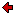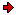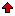Journal of Technical
Physics

A QUARTERLY JOURNALPrevious issue vol. 47, no. 2 (2006) vol. 47, no. 3 (2006)Next issuevol. 47, no. 4 (2006)

### Contents of issue 3, vol. 47

1. B. Singh: Dispersion relations in a generalized monoclinic thermoelastic solid half-space
2. H.A. Attia: Hydromagnetic flow of a dusty fluid between parallel porous plates, considering the ion slip
3. M. Sztyren: Solutions for the higher-grade hybrid model of layered superconductors
4. J.P. Vishwakarma, G. Nath: Converging detonation waves in a dusty gas

B. Singh: Dispersion relations in a generalized monoclinic thermoelastic solid half-space
The plane wave propagation in a homogeneous, monoclinic, generalized thermoelastic solid medium with thermal relaxations is studied. Three types of plane waves: quasi-P (qP), quasi-thermal and quasi-SV (qSV) waves, are shown to exist. The analytical expressions for their dimensional velocities of propagation are obtained. The velocities of these waves are found to depend on the angle of propagation. Angles of reflection for plane waves are found to be different from their angles of incidence. Numerical computations are carried out to show the variations of velocities of plane waves for different parameters. Effects of anisotropy, frequency and thermoelastic coupling coefficient on wave propagation are observed.

ContentsH.A. Attia: Hydromagnetic flow of a dusty fluid between parallel porous plates, considering the ion slip
In the present study, the transient hydromagnetic flow between parallel porous plates of a dusty viscous, incompressible, electrically conducting fluid under the influence of a constant pressure gradient is studied, considering the ion slip. The parallel plates are assumed to be porous and subjected to uniform suction from above and injection from below, while the fluid is acted upon by an external uniform magnetic field applied perpendicular to the plates. The equations of motion are solved analytically to yield the velocity distributions for both the fluid and dust particles as functions of time and space.

ContentsM. Sztyren: Solutions for the higher-grade hybrid model of layered superconductors
A class of basic solutions for a model of layered superconductors is constructed. The considerations are based on the higher-grade hybrid model, constructed in the previous paper. In particular, for any range K of the Josephson interlayer couplings, we determine the spectrum of excited states with respect to all types of ground states calculated there for an infinite uniform superconductor. We discuss also solutions for a finite number of layers with periodic boundary conditions. In this way, instances of the amplitude-modulated solutions for the infinite stack of layers are found.

ContentsJ.P. Vishwakarma, G. Nath: Converging detonation waves in a dusty gas
The problem of converging detonation waves propagating through a dusty gas and releasing a constant amount of energy per unit mass is studied. The dusty gas is assumed to be a mixture of small solid particles and a perfect gas. The solid particles are distributed continuously in the perfect gas. An expression for the similarity exponent is determined for the detonation wave travelling in the Chapman-Jouguet regime. Also, the variation of the flow variables just behind the detonation front with the radius of the front are obtained. It is found that the presence of dust particles in the medium has a significant effect on the similarity exponent and the flow variables.

ContentsReprints of the full papers may be obtained from their authors. Contact Editorial Office in case you need the address of the respective author.
 Home Editors Scope Abstracts For authors Subscription